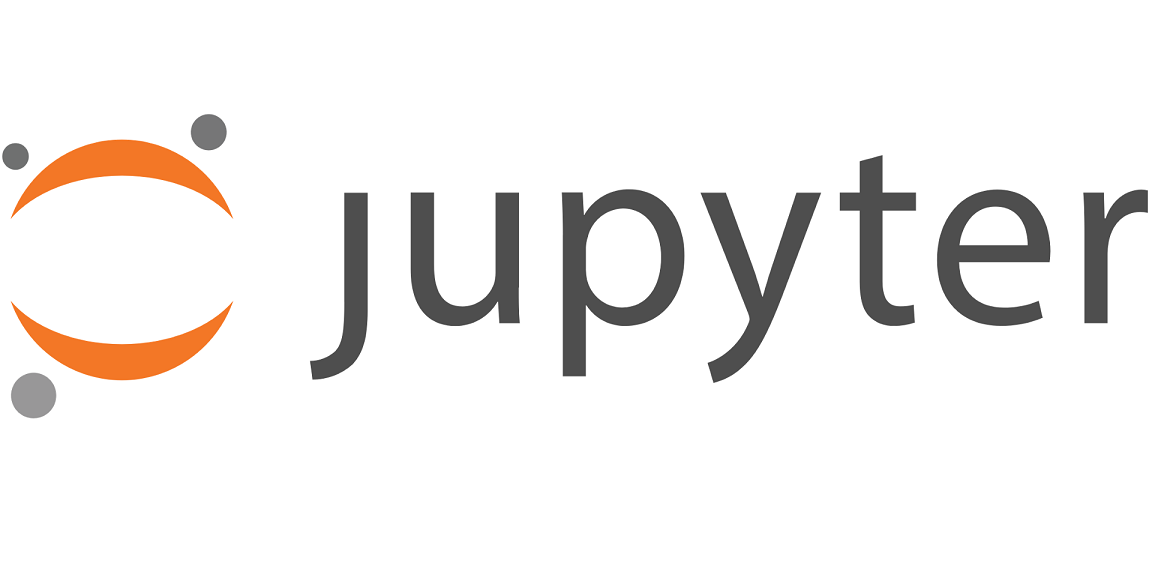Jupyter Notebook 是一个非常强大的工具，常用于交互式地开发和展示数据科学项目。它将代码和它的输出集成到一个文档中，并且结合了可视的叙述性文本、数学方程和其他丰富的媒体。它直观的工作流促进了迭代和快速的开发，使得 notebook 在当代数据科学、分析和越来越多的科学研究中越来越受欢迎。最重要的是，作为开源项目的一部分，它们是完全免费的。

Jupyter 项目是早期 IPython Notebook 的继承者，它在 2010 年首次作为原型发布。尽管在 Jupyter Notebook 中可以使用许多不同的编程语言，但本文将重点介绍 Python，因为在 Jupyter Notebook 中 python 是最常见的。

• 介绍一些安装 Jupyter 和创建你的第一个 notebook 的基本知识。
• 深入钻研，学习所有重要的术语。
• 探索笔记是如何轻松地在网上共享和发布。事实上，这篇文章就是一个 Jupyter notebook！这里的一切都是在 Jupyter notebook 环境中编写的，而你正在以只读的形式查看它。

## 安装

1. 下载支持 Python 3 （就不用 Python 2.7 了）的最新版本 Anaconda。
2. 按照下载页面或可执行文件中的说明安装 Anaconda。

pip3 install jupyter


## notebook 的接口

• 内核是一个“计算引擎”，它执行一个 notebook 文档中包含的代码。
• 单元格是一个容器，用于装载在 notebook 中显示的文本或是会被 notebook 内核执行的代码。

## 单元格

• 代码单元包含要在内核中执行的代码，并在下面显示它的输出。
• Markdown 单元包含使用 Markdown 格式化的文本，并在运行时显示其输出。

print('Hello World!')

Hello World!


import time
time.sleep(3)


def say_hello(recipient):
return 'Hello, {}!'.format(recipient)

say_hello('Tim')

'Hello, Tim!'


## 键盘快捷键

• 在编辑和命令模式之间切换，分别使用 EscEnter
• 在命令行模式下：
• UpDown 键向上和向下滚动你的单元格。
• AB 在活动单元上方或下方插入一个新单元。
• M 将会将活动单元格转换为 Markdown 单元格。
• Y 将激活的单元格设置为一个代码单元格。
• D + D(按两次 D)将删除活动单元格。
• Z将撤销单元格删除。 * 按住 Shift，同时按 UpDown ，一次选择多个单元格。
• 选择了 multple，Shift + M 将合并你的选择。

• Ctrl + Shift + -，在编辑模式下，将在光标处拆分活动单元格。
• 你也可以在你的单元格的左边用 Shift + Click 来选择它们。

## Markdown

Markdown 是一种轻量级的、易于学习的标记语言，用于格式化纯文本。它的语法与 HTML 标记有一对一的对应关系，所以这里的一些经验是有用的，但绝对不是先决条件。请记住，这篇文章是在一个 Jupyter notebook 上写的，所以你所看到的所有的叙述文本和图片都是在 Markdown 完成的。让我们用一个简单的例子来介绍基础知识。

# 这是一级标题。
## 这是一个二级标题。

* 有时我们想要包含列表。
* 可以缩进。

1. 列表也可以编号。
2. 有序列表。

[有可能包括超链接](https://www.example.com)

\
\bar()
\

foo()



• 使用一个在 web 上的图像的 URL。
• 使用一个与你的 notebook 一起维护的本地 URL，例如在同一个 git 仓库中。
• 通过 "Edit > Insert Image" 添加附件；这将把图像转换成字符串并存储在你的 notebook 中的 .ipynb 文件。
• 注意这将使你的 .ipynb 的文件更大!

Markdown 有很多细节，特别是在超链接的时候，也可以简单地包括纯 HTML。一旦你发现自己突破了上述基础的限制，你可以参考 Markdown 创造者 John Gruber 的官方指南

## 内核

import numpy as np

def square(x):
return x * x


x = np.random.randint(1, 10)
y = square(x)

print('%d squared is %d' % (x, y))

1 squared is 1


print('Is %d squared is %d?' % (x, y))

Is 1 squared is 1?


y = 10


• 重启：重新启动内核，从而清除定义的所有变量。
• 重启和清除输出:与上面一样，但也将擦除显示在您的代码单元格下面的输出。
• 重启和运行所有:和上面一样，但也会运行你的所有单元，从第一个到最后。

## 设置

%matplotlib inline

import pandas as pd
import matplotlib.pyplot as plt
import seaborn as sns

sns.set(style="darkgrid")


df = pd.read_csv('fortune500.csv')


## 调查我们的数据集

df.head()


YearRankCompanyRevenue (in millions)Profit (in millions)019551General Motors9823.5806119552Exxon Mobil5661.4584.8219553U.S. Steel3250.4195.4319554General Electric2959.1212.6419555Esmark2510.819.1

df.tail()


YearRankCompanyRevenue (in millions)Profit (in millions)254952005496Wm. Wrigley Jr.3648.6493254962005497Peabody Energy3631.6175.4254972005498Wendy's International3630.457.8254982005499Kindred Healthcare3616.670.6254992005500Cincinnati Financial3614.0584

df.columns = ['year', 'rank', 'company', 'revenue', 'profit']


len(df)

25500


df.dtypes

year         int64
rank         int64
company     object
revenue    float64
profit      object
dtype: object


non_numberic_profits = df.profit.str.contains('[^0-9.-]')


yearrankcompanyrevenueprofit2281955229Norton135.0N.A.2901955291Schlitz Brewing100.0N.A.2941955295Pacific Vegetable Oil97.9N.A.2961955297Liebmann Breweries96.0N.A.3521955353Minneapolis-Moline77.4N.A.

set(df.profit[non_numberic_profits])

{'N.A.'}


len(df.profit[non_numberic_profits])

369


bin_sizes, _, _ = plt.hist(df.year[non_numberic_profits], bins=range(1955, 2006))


df = df.loc[~non_numberic_profits]
df.profit = df.profit.apply(pd.to_numeric)


len(df)

25131

df.dtypes

year         int64
rank         int64
company     object
revenue    float64
profit     float64
dtype: object


## 使用 matplotlib 进行绘图

group_by_year = df.loc[:, ['year', 'revenue', 'profit']].groupby('year')
avgs = group_by_year.mean()
x = avgs.index
y1 = avgs.profit

def plot(x, y, ax, title, y_label):
ax.set_title(title)
ax.set_ylabel(y_label)
ax.plot(x, y)
ax.margins(x=0, y=0)


fig, ax = plt.subplots()
plot(x, y1, ax, 'Increase in mean Fortune 500 company profits from 1955 to 2005', 'Profit (millions)')


y2 = avgs.revenue
fig, ax = plt.subplots()
plot(x, y2, ax, 'Increase in mean Fortune 500 company revenues from 1955 to 2005', 'Revenue (millions)')


def plot_with_std(x, y, stds, ax, title, y_label):
ax.fill_between(x, y - stds, y + stds, alpha=0.2)
plot(x, y, ax, title, y_label)

fig, (ax1, ax2) = plt.subplots(ncols=2)
title = 'Increase in mean and std Fortune 500 company %s from 1955 to 2005'
stds1 = group_by_year.std().profit.as_matrix()
stds2 = group_by_year.std().revenue.as_matrix()
plot_with_std(x, y1.as_matrix(), stds1, ax1, title % 'profits', 'Profit (millions)')
plot_with_std(x, y2.as_matrix(), stds2, ax2, title % 'revenues', 'Revenue (millions)')
fig.set_size_inches(14, 4)
fig.tight_layout()


## 在你分享之前

1. 点击 "Cell > All Output > Clear"
2. 点击 "Kernel > Restart & Run All"
3. 等待您的代码单元完成执行，并检查它们是否按预期执行。

## 导出你的 notebook

Jupyter 内置支持导出 HTML 和 PDF 以及其他几种格式，你可以在 File > Download As 菜单下找到。如果你希望与一个小型的私有组共享你的 notebook，这个功能很可能是你所需要的。事实上，许多学术机构的研究人员都有一些公共或内部的网络空间，因为你可以将一个 notebook 导出到一个 HTML 文件中，Jupyter notebook 可以成为他们与同行分享成果的一种特别方便的方式。

## GitHub

1. 登录并且浏览 gist.github.com
2. 用文件编辑器打开 .ipynb 文件, 全选并且拷贝里面的 JSON 。
3. 将笔记的 JSON 粘贴到中 gist 中。
4. 给你的 Gist 命名, 记得添加 .iypnb 后缀，否则不能正常工作。
5. 点击 "Create secret gist"或者 "Create public gist."

NBViewer 显示的 notebook 的 URL 是基于正在渲染的 notebook 的 URL 的并且不会改变，所以你可以和任何人分享它，只要原始文件保持在线 —— NBViewer 不会缓存文件很长时间。